## Example Questions

### Example Question #1 : How To Subtract Negative Numbers

What is 1 + (–1) – (–3) + 4 ?

9

7

6

3

1

7

Explanation:

You simplify the expression to be 1 – 1 + 3 + 4 = 7

### Example Question #1 : Negative Numbers

Solve the following equation for: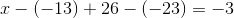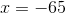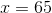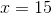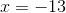Explanation:

To begin, we need to recall how to subtract negative numbers. Remember, when subtracting a negative number, the two negatives cancel out, creating a positive.

So, this:Becomes this: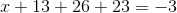We can combine like terms on the left to get: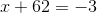Then, we can subtract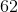from both sides in order to getby itself: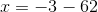In this case, we are subtracting from a negative number, which is just like adding two negative numbers, or subtracting from a positive number. The result will be more negative, because we will be moving further to the left on the number line.### Example Question #1 : Describe Situations In Which Opposite Quantities Combine To Make 0: Ccss.Math.Content.7.Ns.A.1a

Compute the following: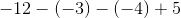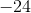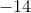Explanation:

Convert all the double signs to a single sign before solving. Remember, two minus (negative) signs combine to form a plus (positive) sign, and a plus (positive) sign and a minus (negative) sign combine to form a minus (negative) sign.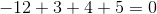### All ACT Math Resources